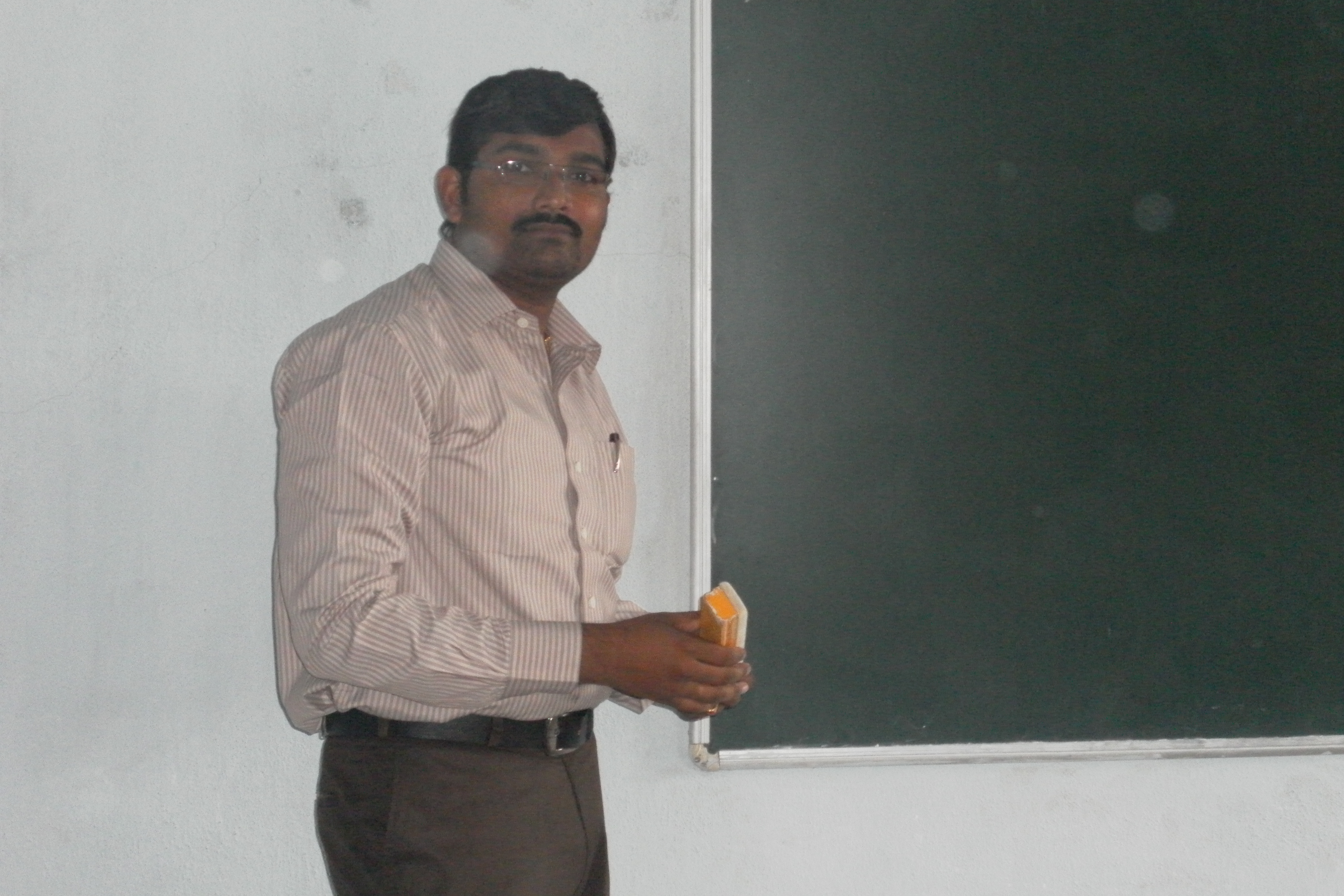Search
•CH.PHYSICS

# CBSE Class 12 Physics Practical Exam Question Paper

Updated: Apr 26, 2020

## AISSCE (PRACTICAL) CLASS – XII – 2020

Subject: PHYSICS (042) Date: __,__, 2020.

COLLEGE / SCHOOL NAME, (COLLEGE CODE) Time: 3 hr.

## QUESTION PAPER

SECTION – A 1 X 8M = 8M

1. To determine resistance per cm of a given wire by plotting a graph of potential difference versus current.

2. To verify the law of combination of series of resistances using a meter bridge.

3. To verify the law of combination of parallel of resistances using a meter bridge.

4. To determine the internal resistance of a given primary cell using potentiometer.

5. To find resistance of a given wire using meter bridge and hence determine the specific resistance of its material.

6. To determine the frequency of AC using sonometer.

7. To compare the emf of given cells using potentiometer.

8. To determine the internal resistance of a given galvanometer and find its figure of merit.

SECTION – B 1 X 8M = 8M

9. To find the focal length of a concave mirror by plotting graph between u and v.

10. To find the focal length of a convex lens by plotting graph between u and v.

11. To find the focal length of a convex mirror using a convex lens.

12. To find the refractive index of a liquid by using convex lens and plane mirror.

13. To draw the V-I characteristic curve of a p-n junction diode in forward bias and reverse bias.

14. To draw the V-I characteristic curve of a Zener diode and to determine its reverse breakdown voltage.

15. To determine refractive index of material of the prism by measuring angle of minimum deviation.

16. To draw the input and output characteristics of a NPN transistor in common emitter configuration.

SECTION – C

Record and Activities record ------------------ 6M

Project ------------------ 3M

Viva (On Practical, activities and project) ---------- 5M

TOTAL MARKS ---------- 30M